# Oguzhan Teke - Research

My research can be categorized as follows:

# Random Asynchronous Linear Systems

Linear state-space recursions arise in a large number of different fields ranging from mathematical finance to implementation of digital filters. Such recursions are also used commonly as power methods in numerical linear algebra, which provide useful tools for data analysis. A notable application is the PageRank algorithm used in search engines for ranking web pages. More recently, state-space models are studied also in the analysis of network defined data, where the state vector is considered as a signal on the graph, and the transition matrix is considered as a local graph operator that encapsulates the connectivity structure of the underlying network. Then, a state recursion can be implemented on the graph in a distributed fashion as a data exchange between the neighboring nodes.

In more practical scenarios, nodes on the graph can communicate with each other asynchronously and randomly. That is, a single node may “wake up” at a random time instance, do some local computations on its own, and then send information to its outgoing neighbors.Such random and asynchronous behavior of the nodes can be modeled with the following asynchronous state recursions: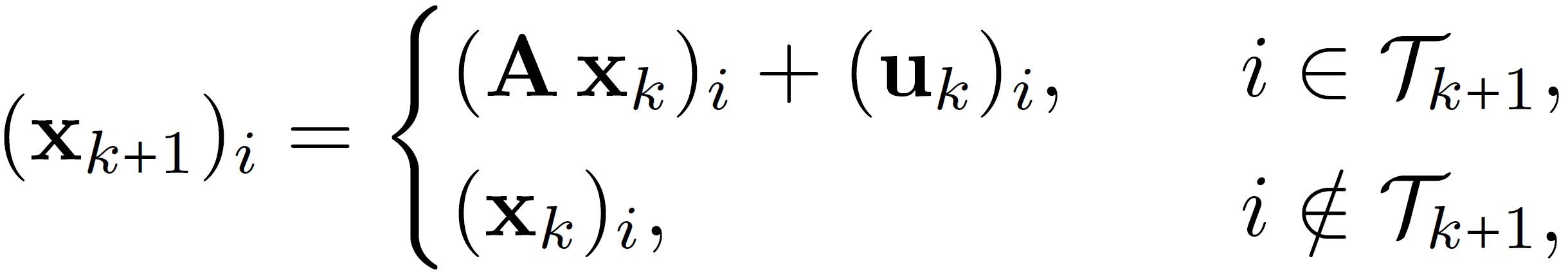which is very similar to the classical (synchronous) state recursions except the fact that only a random subset of variables are updated at an iteration, and the remaining ones stay unchanged.

Since the dynamical behavior of randomized asynchronous updates is very different from standard state-space models, in this line work we have focused on the convergence and stability behavior of such random asynchronous recursions. In particular we have shown that an unstable system (in the synchronous world) may be stabilized simply by the use of randomized asynchronicity. Moreover, randomized asynchronicity may result in a lower total computational complexity in some applications.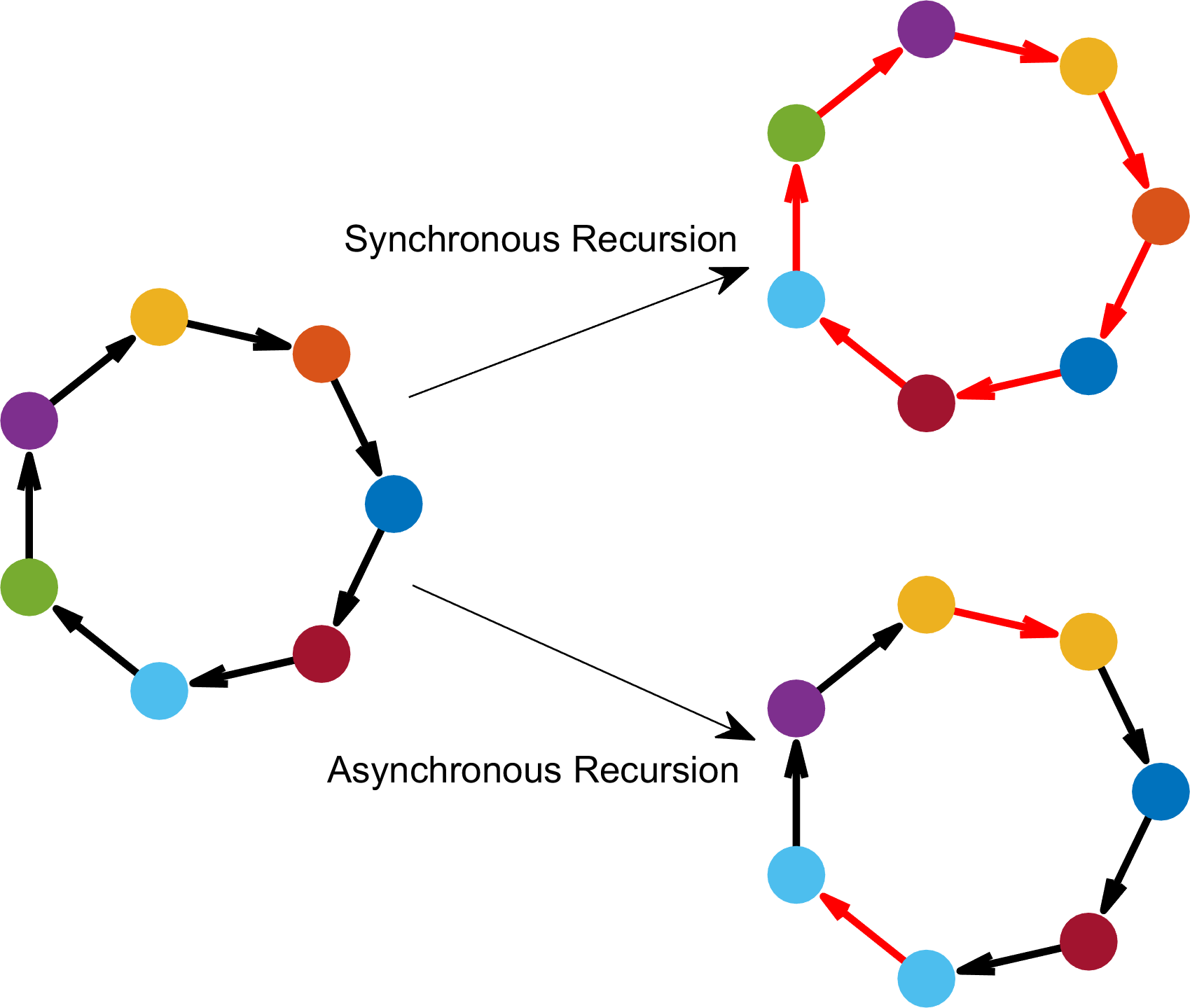Demonstration of asynchronous and synchronous recursions on the directed cycle graph of size N = 7. When all the nodes “fire” synchronously, the graph signal is shifted circularly. So, the signal repeats itself after every N synchronous updates. When only a random subset of nodes fire asynchronously, duplicate entries are created, and the signal converges (in the mean-squared sense) to a constant signal on the graph eventually with random asynchronous updates.

### Related Publications

O. Teke, P. P. Vaidyanathan, "Random Asynchronous Linear Systems: Frequency Response Behavior," IEEE Transactions on Signal Processing, in preparation.
O. Teke, P. P. Vaidyanathan, "Random Node-Asynchronous Graph Computations”, IEEE Signal Processing Magazine, submitted as a white paper, Oct. 2019.
O. Teke, P. P. Vaidyanathan, "IIR Filtering on Graphs with Random Node-Asynchronous Updates," IEEE Transactions on Signal Processing, under review, June 2019.
[J8] O. Teke, P. P. Vaidyanathan, "Random Node-Asynchronous Updates on Graphs," IEEE Transactions on Signal Processing, vol. 67, no. 11, pp. 2794-2809, June 2019. [pdf]
[C18] O. Teke, P. P. Vaidyanathan, "Node-Asynchronous Spectral Clustering on Directed Graphs," Int. Conf. Acoust. Speech, Signal Process. (ICASSP), submitted, 2020. [pdf]
[C17] O. Teke, P. P. Vaidyanathan, "Randomized Asynchronous Recursions with a Sinusoidal Input," Asilomar Conference on Signals, Systems, and Computers, to appear, 2019. [pdf]
[C16] O. Teke, P. P. Vaidyanathan, "The random component-wise power methods," Proc. SPIE, Wavelets and Sparsity XVIII, vol. 11138, Sep. 2019. [pdf]
[C15] O. Teke, P. P. Vaidyanathan, "Node-asynchronous Implementation of Rational Filters on Graphs,” Int. Conf. Acoust. Speech, Signal Process. (ICASSP), pp. 7530-7534, May 2019. [pdf]
[C13] O. Teke, P. P. Vaidyanathan, "Asynchronous Nonlinear Updates on Graphs," Asilomar Conference on Signals, Systems, and Computers, pp. 998–1002, Oct. 2018. [pdf]
[C12] O. Teke, P. P. Vaidyanathan, "The Asynchronous Power Iteration: A Graph Signal Perspective," Int. Conf. Acoust. Speech, Signal Process. (ICASSP), pp. 4059-4063, April 2018. [pdf] [slides]

# Sparsity and Uncertainty on Graphs

The uncertainty principle is an essential mathematical concept in science and engineering, and it explains some fundamental limits in different areas ranging from quantum mechanics to harmonic analysis. In classical signal processing, the uncertainty principle states that a signal cannot be arbitrarily localized in time and frequency simultaneously. More generally, uncertainty principles state that a signal cannot have an arbitrarily "short" description in two different bases simultaneously. Due to its fundamental characterization, uncertainty principles have been useful to design signals, pulse shapes, and filters that are maximally localized in one domain for a given spread in the other domain. Furthermore, they lay a foundation for sparse representation theory and compressed sensing.

Since graph signal processing proposes two different bases (i.e., vertex and the graph Fourier domains) to represent graph signals, my research in this context considers the localization properties of graph signals in these two domains. In particular, I have focused on discrete uncertainty principles based on the notion of sparsity. I have shown that the total number of nonzero elements of a graph signal and its representation in the graph Fourier domain (i.e., graph Fourier transform) is lower bounded by a quantity depending on the underlying graph. In the particular case of directed cycle graph (which relates the graph signal processing to classical signal processing), I have also shown that the proposed inequalities reduce to the well-known uncertainty principles in discrete signal processing.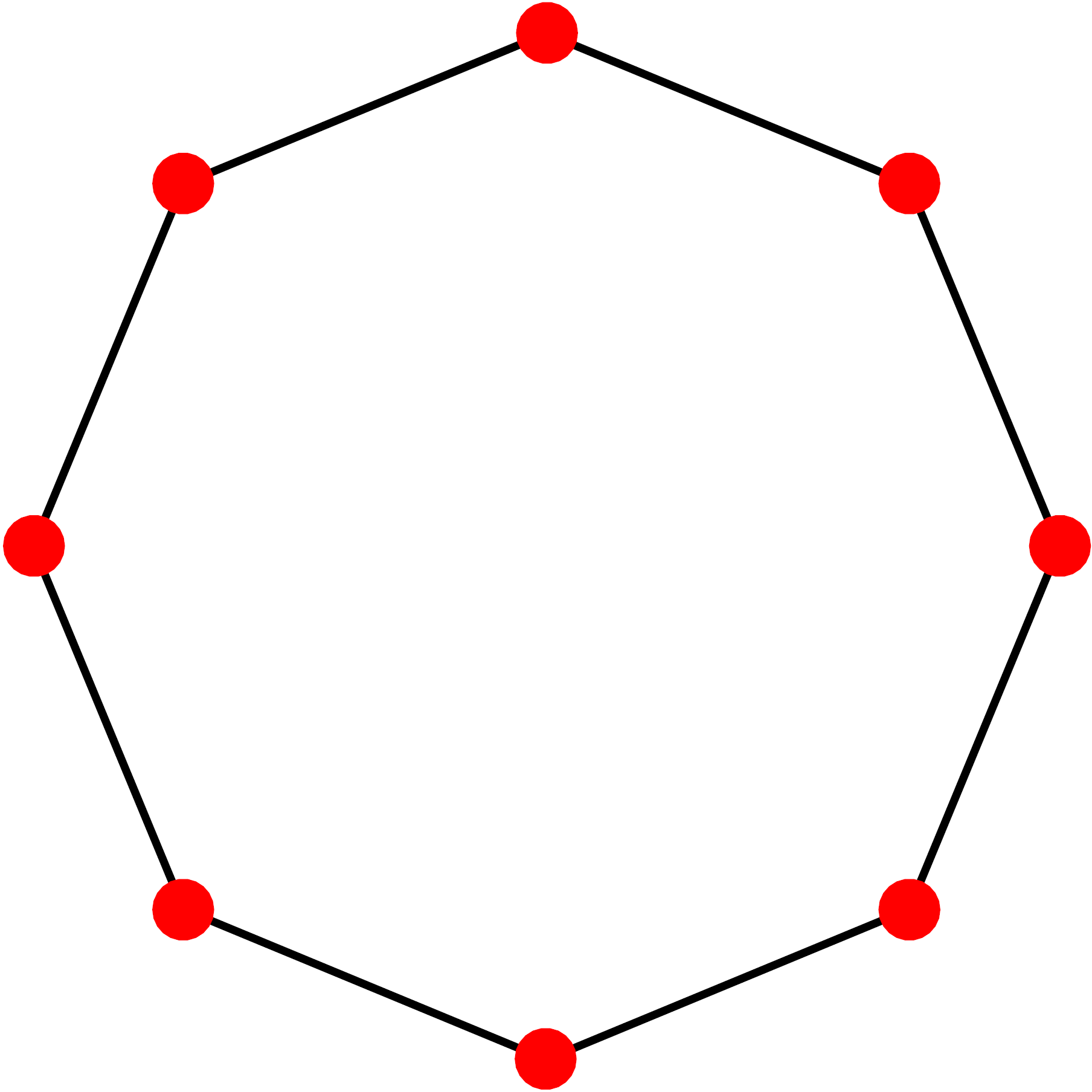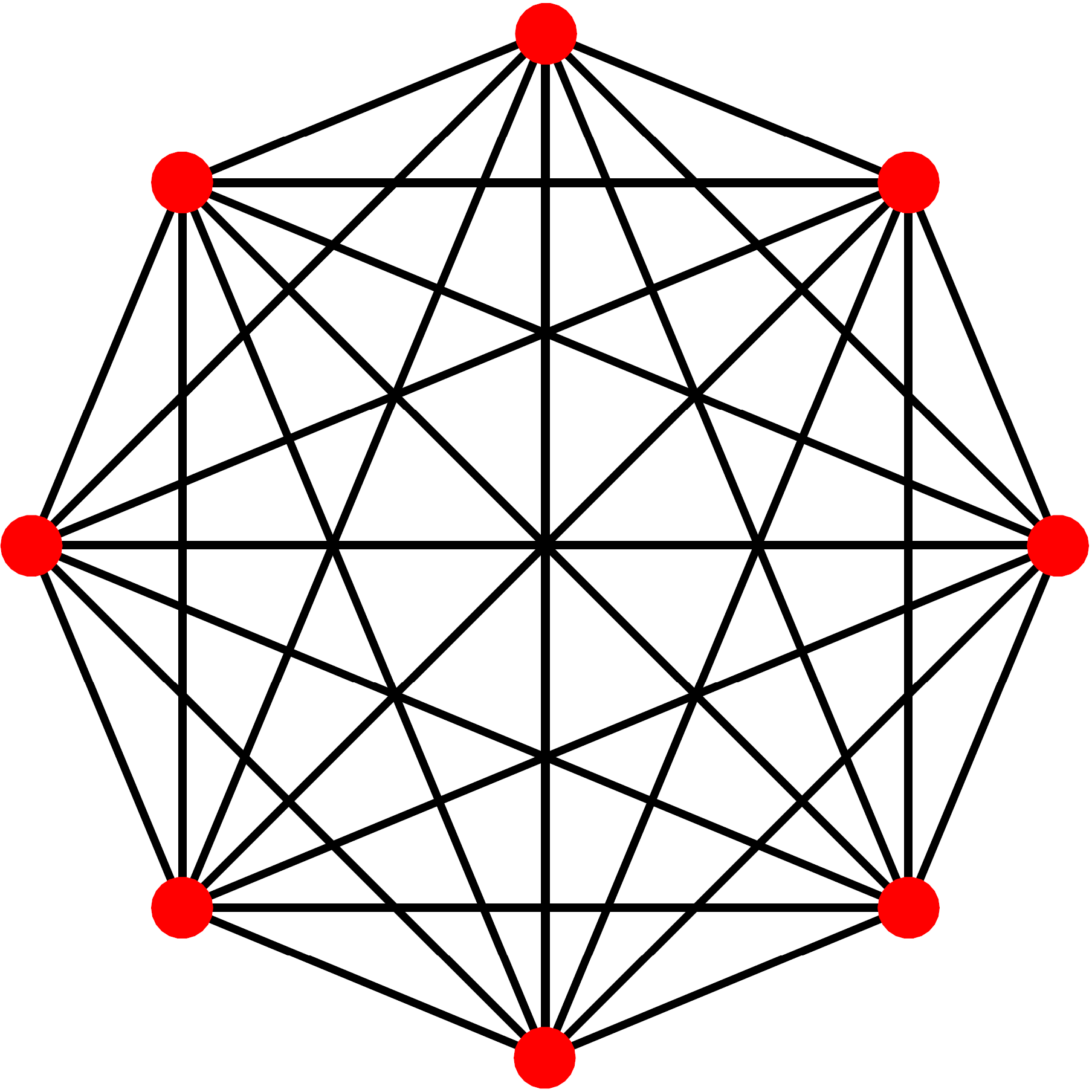An eigenvector of a cycle graph of size N has at least N/2 nonzero entries, whereas a complete graph has 2-sparse eigenvectors. Thus, signals on a complete graph can be extremely localized in vertex and graph Fourier domains simultaneously.

### Related Publications

[J7] O. Teke, P. P. Vaidyanathan, "Uncertainty principles and sparse eigenvectors of graphs," IEEE Transactions on Signal Processing, vol. 65, no. 20, pp. 5406-5420, Oct. 2017. [pdf]
[C09] O. Teke, P. P. Vaidyanathan, "Sparse Eigenvectors of Graphs," Int. Conf. Acoust. Speech, Signal Process. (ICASSP), pp. 3904-3908, March 2017. [pdf] [slides]
[C07] O. Teke, P. P. Vaidyanathan, "Discrete Uncertainty Principles on Graphs," Asilomar Conference on Signals, Systems, and Computers, pp. 1475-1479, Nov. 2016. [pdf]

# Multirate Processing of Graph Signals

Multirate systems and filter banks find many applications in signal processing theory and implementations. Due to its significance and applicability to wide range of problems, extensions of classical multirate signal processing ideas to the case of graphs became a popular sub-field in graph signal processing. The first study showed the possibility of extending 2-channel filter banks to bipartite graphs, as their eigenvalue spectrum has a symmetric structure. However, this relation cannot be generalized to M-channel systems on M-partite graphs. As a result, the extension of classical multirate theory to graphs is nontrivial, and such extensions cannot be obtained without certain mathematical restrictions on the graph. For example, even a simple delay chain system does not provide perfect reconstruction (PR) on an arbitrary graph (as apposed to classical filter banks). My research in this context considered the extension of fundamental building blocks of multirate signal processing theory to the case of graphs . I revisited ideas such as noble identities, aliasing, and polyphase decompositions in graph multirate systems. I provided the necessary conditions on the graph such that these ideas remain valid in the graph domain. In particular, I showed that when the underlying graph satisfies a condition called M-block cyclic property, classical multirate theory can be extended to the graphs.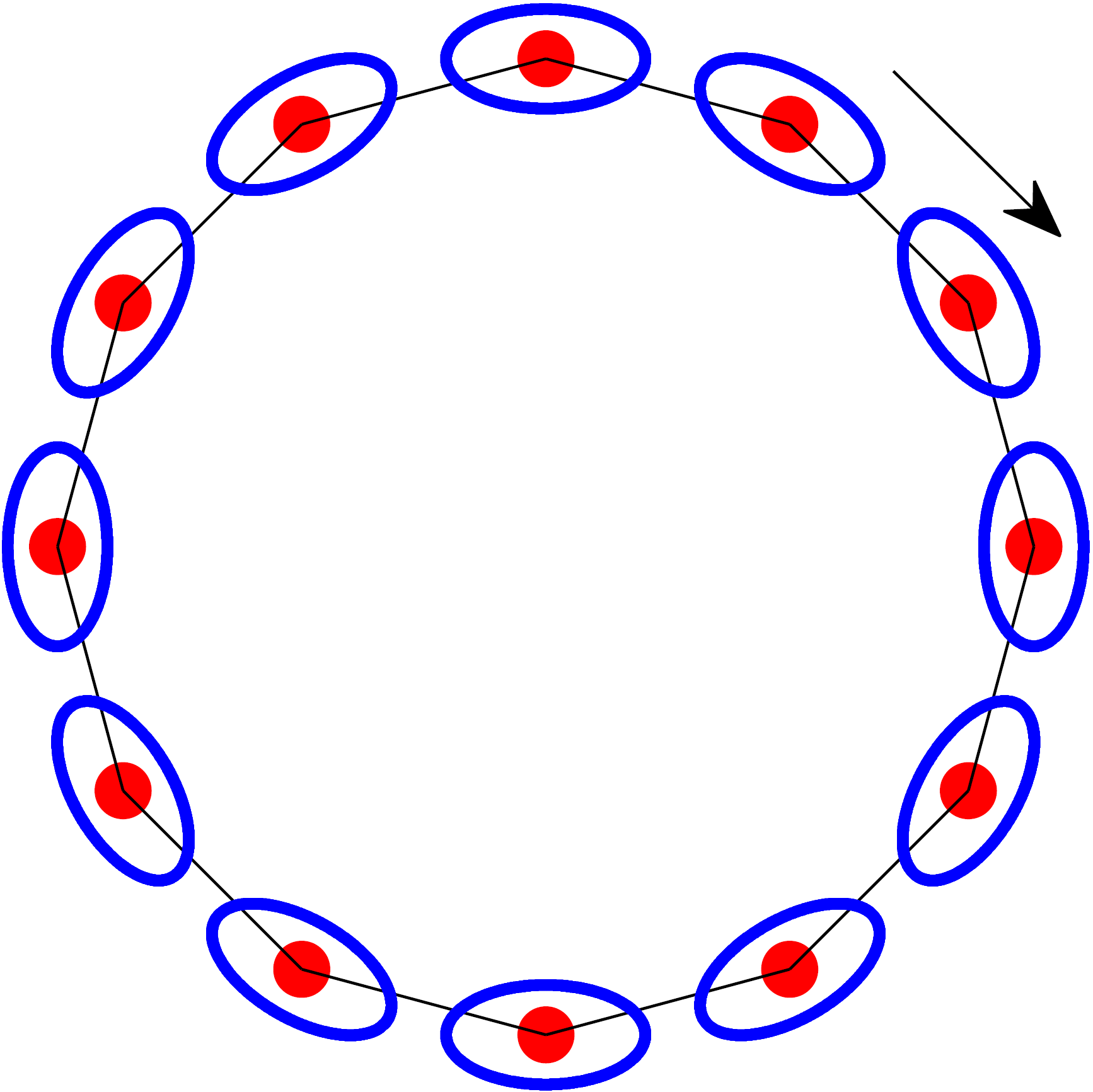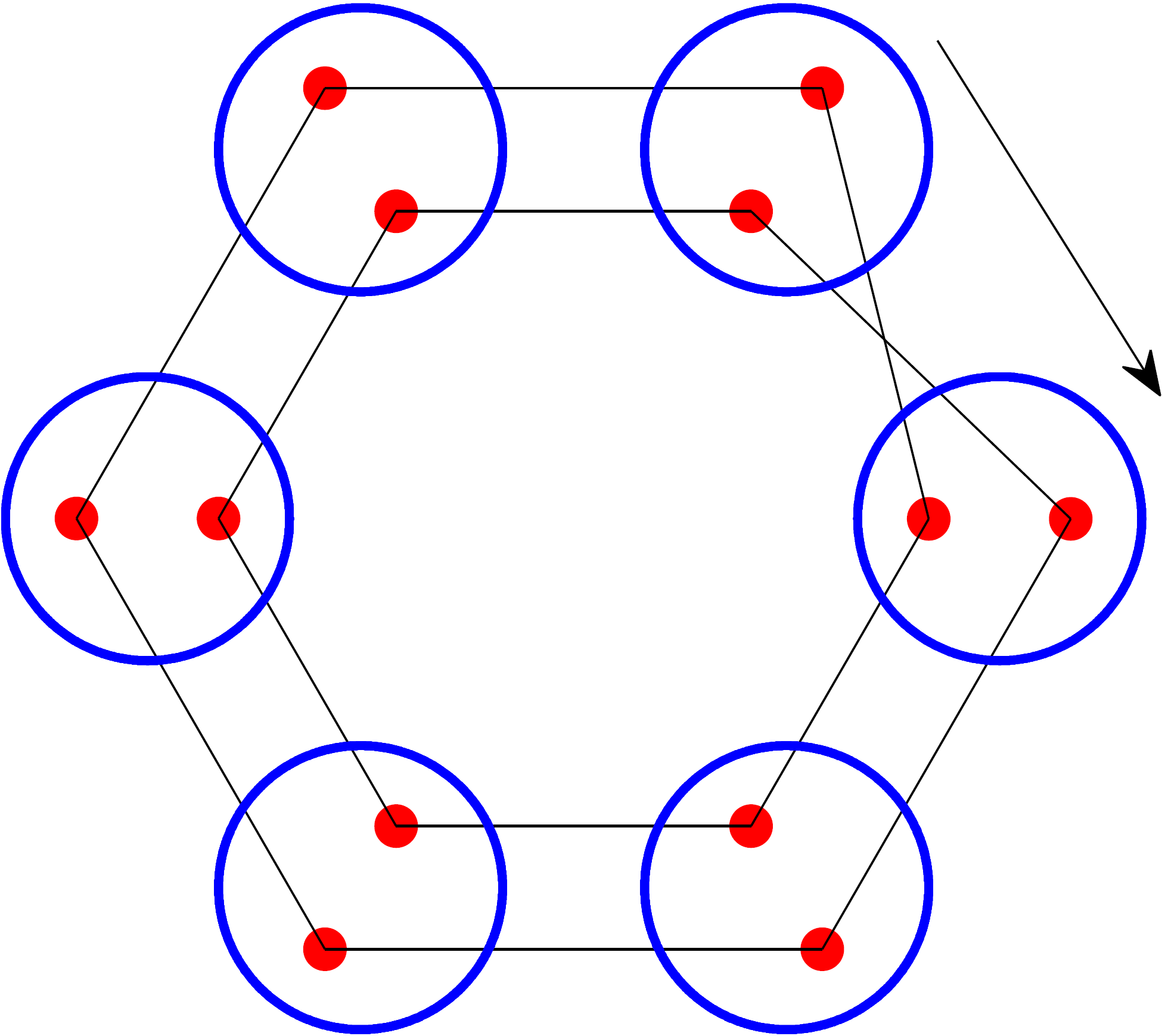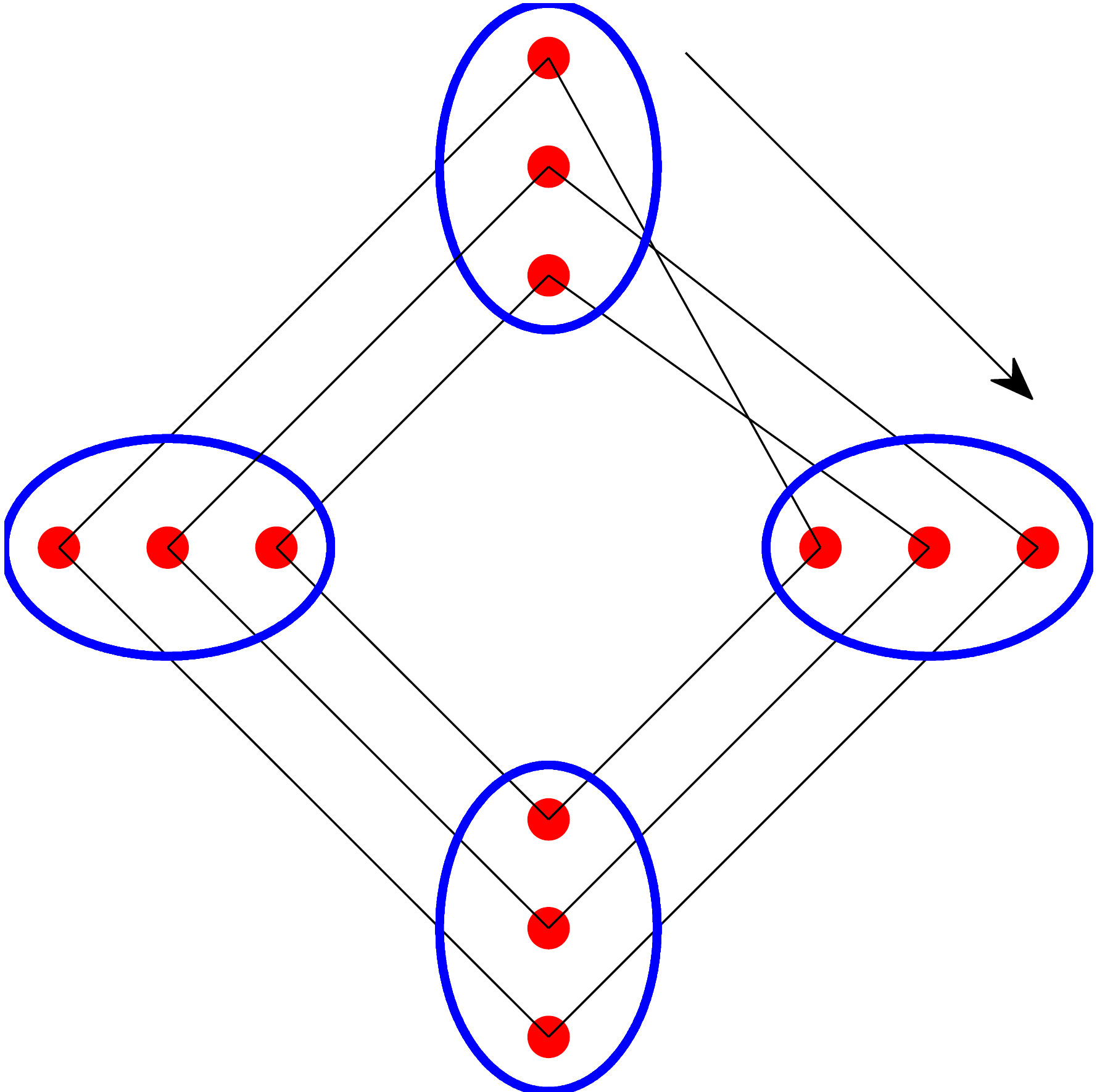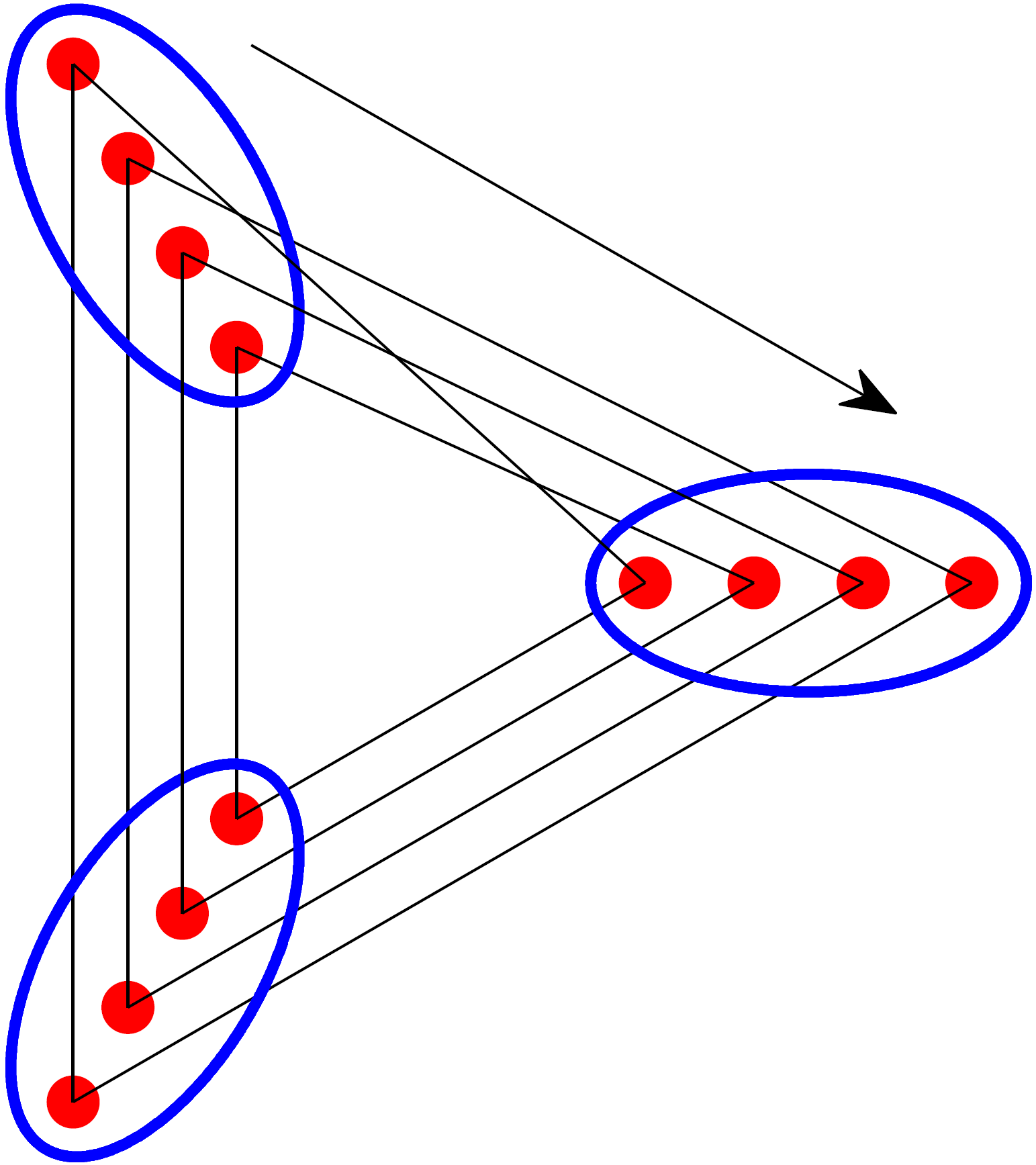In general, M-block cyclic property is more restrictive than M-partiteness. Nevertheless, a directed cycle graph of size N, CN, (which relates graph signal processing to classical signal processing) can be represented as an M-block cyclic graph under suitable permutation of the vertices.

### Related Publications

[J5] O. Teke, P. P. Vaidyanathan, "Extending Classical Multirate Signal Processing Theory to Graphs - Part I: Fundamentals," IEEE Transactions on Signal Processing, vol. 65, no. 2, pp. 409-422, Jan. 2017. [pdf]
[J4] O. Teke, P. P. Vaidyanathan, "Extending Classical Multirate Signal Processing Theory to Graphs - Part II: M-Channel Filter Banks," IEEE Transactions on Signal Processing, vol. 65, no. 2, pp. 423-437, Jan. 2017. [pdf]
[C10] O. Teke, P. P. Vaidyanathan, "Extending classical multirate signal processing theory to graphs," Proc. SPIE, Wavelets and Sparsity XVII, vol. 10394, Aug. 2017. [pdf]
[C06] O. Teke, P. P. Vaidyanathan, "Graph filter banks with M-channels, maximal decimation, and perfect reconstruction," Int. Conf. Acoust. Speech, Signal Process. (ICASSP), pp. 4089-4093, March 2016.[pdf]
[C05] O. Teke, P. P. Vaidyanathan, "Fundamentals of multirate graph signal processing," Asilomar Conference on Signals, Systems, and Computers, pp. 1791-1795, Nov. 2015. [pdf]

# Off-grid Problem in Compressed Sensing

Prior to my doctoral work at California Institute of Technology, I studied for M.S. degree where my research focused on robust sparse recovery techniques in the presence of a basis mismatch due to the discretization of the underlying continuous parameter space. Another way to tackle this issue is to formulate an atomic norm minimization problem using the continuous dictionary itself. In the case of line spectral estimation, the atomic norm minimization problem can be written as semi-definite programming using tractable representations of nonnegative trigonometric polynomials. As a part of my doctoral work, I have developed an alternative proof for the SDP representation of atomic norm problems. This is a standalone proof that relies only on fundamental results from matrix theory, and it shows that the bounded lemma from system theory literature directly leads to the SDP formulations.

### Related Publications

O. Teke, "Robust Compressive Sensing Techniques," M.S. thesis, Department of EEE, Bilkent University, Turkey, July 2014. [pdf]
[J6] O. Teke, P. P. Vaidyanathan, "On the Role of the Bounded Lemma in the SDP Formulation of Atomic Norm Problems," IEEE Signal Processing Letters, vol. 24, no. 7, pp. 972-976, July 2017. [pdf] [poster]
[J3] O. Teke, A. Gurbuz, O. Arikan, "A Robust Compressive Sensing Based Technique For Reconstruction of Sparse Radar Scenes," Digital Signal Processing, vol. 27, pp. 23-32, April 2014. [pdf]
[J2] O. Teke, A. Gurbuz, O. Arikan, "Perturbed orthogonal matching pursuit," IEEE Transactions on Signal Processing, vol. 61, no. 24, pp. 6220-6231, Dec. 2013. [pdf]
[J1] A. Gurbuz, O. Teke, O. Arikan, "Sparse ground-penetrating radar imaging method for off-the-grid target problem," Journal of Electronic Imaging, vol. 22, no. 2, pp. 1-8, 2013. [pdf]
[C04] O. Teke, A. Gurbuz, O. Arikan, "A recursive way for sparse reconstruction of parametric spaces," Asilomar Conference on Signals, Systems, and Computers, pp. 637-641, Nov. 2014. [pdf]
[C03] O. Teke, A. Gurbuz, O. Arikan, "Sparse delay-doppler image reconstruction under off-grid problem," Sensor Array and Multichannel Signal Processing Workshop (SAM), pp. 409-412, June 2014. [pdf]
[C02] O. Teke, A. Gurbuz, O. Arikan, "Sparse Reconstruction Under Model Uncertainties," Signal Processing with Adaptive Sparse Structured Representations (SPARS), July 2013. [pdf]